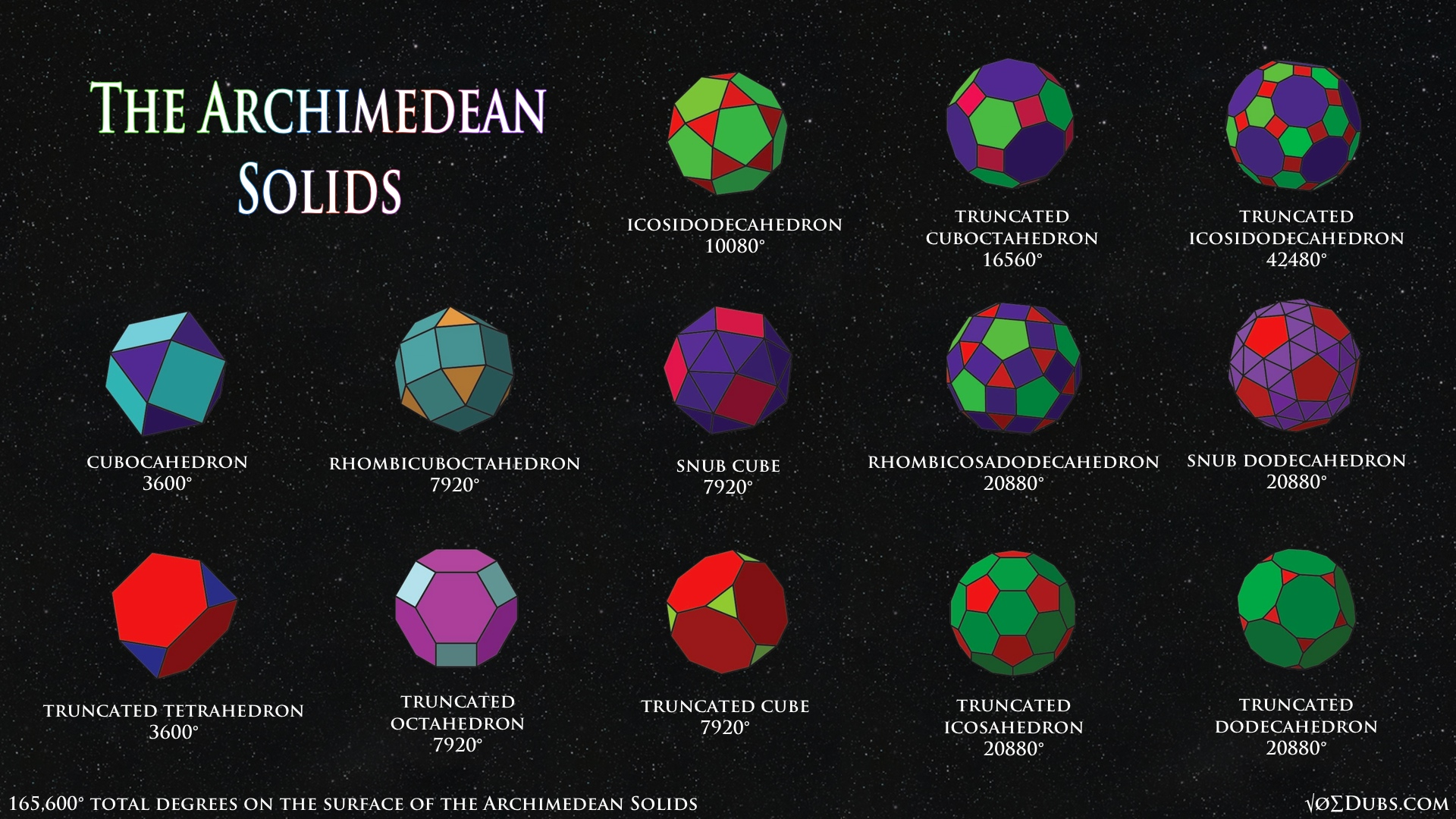## The Archimedean Solids & Their Dual Catalan SolidsThe Archimedean solids and their duals the Catalan solids are less well known than the Platonic solids.  Whereas the Platonic solids are composed of one shape, these forms that Archimedes wrote about are made of at least two different shapes, all forming identical vertices.  They are 13 polyhedra of this type.  And since each solid has a ‘dual’ there are also 13 Catalan solids.  There are 26 in total.  If  our two-dimensional letters of the alpha-bet were three dimensional forms, these solids would be a great representation of our language.

3

## The Divine Proportion: Golden (Phi)nomena of NatureThere is one proportion that is divine.  A ratio of two numbers, the simplest of which being one.  Paired with unity, there is only one number that is capable of fractal expansion and contraction.  By using the four operations of mathematics: addition, subtraction, multiplication, and division, this golden number (1.6180339..) is the key to understanding fractality, holograms, life, and the nature of reality.  This is the number (or ratio) the universe uses to become more aware of itself. The divine proportion is the only mathematical ratio that the Universe needs to intelligently design all life.  Phi, aka the Golden Ratio,  is a truly divine number.  It’s quite a phinomenon.  Along with unity this number creates the perfect proportion.  Let’s demystiphi some of the more esoteric geometric concepts attributed to this golden proportion.   Phi is found everywhere and yet nobody talks about it.  It’s missing from the calculator.  Its…

12

## Vitruvian Man HandledLeonardo da Vinci’s famous depiction of Vitruvius encodes geometrical gnosis that’s hidden deep beneath the collective unconscious. Let’s man handle these esoteric concepts and bring light to where there is darkness Leonardo was familiar with the golden ratio and modeled Vitruvian’s square and circle off it. It turns out that this Phi ratio is almost exactly smack dab in the middle of the two solutions of ‘squaring the circle’, that is drawing a square that is commensurate to a circle, either by having equal areas, or equal perimeters ( or lengths).

6